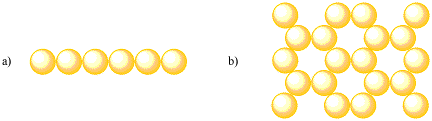# E. Problems

### Problem ME2.1.

1. Look at the simple square layer. Can you find repeating patterns in other directions (rather than left-right or front-back)? If so, in what direction?

2. Look at the hexagonal layer. Can you find repeating patterns in other directions (rather than left-right or front-back)? If so, in what direction?

### Problem ME2.2.

We usually think of atoms as little spheres, or circles if we are working in just two dimensions. How might other shapes form an organized layer? Draw an organized layer for each of the following shapes, using about a dozen units in each case.

1. squares.
2. triangles (equilateral: all sides the same length).
3. hexagons.
4. pentagons.

In each case, is there only one way to pack the shapes in a regular way? Can you find repeating patterns in the layer? If so, in what direction?

Are there any shapes that seemed more difficult to arrange in a repeating layer?

### Problem ME2.3.

Holes generally have particular shapes. The shapes vary, depending on how the atoms are arranged. For example, how would you describe the shape of the holes in the following layers:

1. simple square packing.
2. hexagonal or close packing.

You can come up with an answer by looking at a drawing, or you can try packing a layer of dimes or pennies or quarters (as long as they are all the same) and look at the spaces between them.

### Problem ME2.4.

In the following cases, show that the given unit cells repeat throughout the structure.

1. simple square layer: a square unit cell
2. hexagonal layer: a rhombic unit cell

Note: in the hexagonal layer, you can find an additional way to do this if you rotate copies of the unit cell to get unit cells facing in three different directions.

### Problem ME2.5.

In the following cases, show what fractions of atoms are found in the unit cell. Add the fractions to find the combined total of atoms in the unit cell.

1. simple square layer: a square unit cell
2. hexagonal layer: a rhombic unit cell

### Problem ME2.6.

Go to your piggy bank and break out a bunch of pennies, nickels, quarters or dimes. You will need at least four of each, but nine of them is a good number. Pack one type of coin into the following layers:

1. simple square
2. hexagonal or close packed

In each case, measure the distance from the edge of one coin to the center of the hole.

In which case is the free electron closer to the atom? Does the packing system (the type of layer) have an effect on how tightly bonded that atoms are?

### Problem ME2.7.

Go to your piggy bank and break out a bunch of quarters AND a bunch of dimes. You will need at least four of each, but nine of them is a good number. Pack each type of coin into the following layers:

1. simple square
2. hexagonal or close packed

In each case, measure the distance from the edge of one coin to the center of the hole.

In which case is the free electron closer to the atom? Does the size of the atom have an effect on how tightly bonded that atoms are?

### Problem ME2.8.

When a metal melts, the atoms go from a tightly-bound, regular array of atoms to a loosely-bound set of mobile atoms. Compare the melting points of the following metals, given in degrees Celsius.

potassium: 64 cesium: 29 lithium 181 sodium: 98 francium: 27

1. Can you determine any trends among these values?
2. Can you provide a physical explanation for your observation? (You may have found a trend, but can you explain it?)

### Problem ME2.9.

Packing efficiency is often determined more rigorously in terms of the percentage of a unit cell that is actually occupied by atoms. What is the total area of a unit cell in the following layers?

1. simple square layer.
2. hexagonal layer.

You can assume the atoms are titanium. Titanium has an atomic radius of 2.00 Angstroms (or 2.00 x 10-10 m).

### Problem ME2.10.

How many titanium atoms are there in a unit cell in the following layers?

1. simple square layer.
2. hexagonal layer.

You may need to add up fractions of titanium atoms to arrive at the answer. The answer may or may not be a whole number.

### Problem ME2.11.

What is the area occupied by a titanium atom?

(If you think the question is ambiguous, assume we're concerned about the area of a cross-section of the atom at its widest part. It is a spherical atom, but we are dealing with its projection in two dimensions, i.e. its shadow when the sun is directly overhead.)

### Problem ME2.12.

In the following cases, what percentage of the unit cell is filled with titanium atoms?

1. simple square layer.
2. hexagonal layer.

The percentage of unit cell that is occupied by atoms is called "the packing efficiency".

### Problem ME2.13.

What is the coordination number of an atom in

1. simple square layer
2. hexagonal layer

### Problem ME2.14.

What is the coordination number of an atom in the following layers:### Problem ME2.15.

What is the coordination geometry of an atom in

1. simple square layer
2. hexagonal layer

### Problem ME2.16.

What is the coordination geometry of an atom in the following layers: